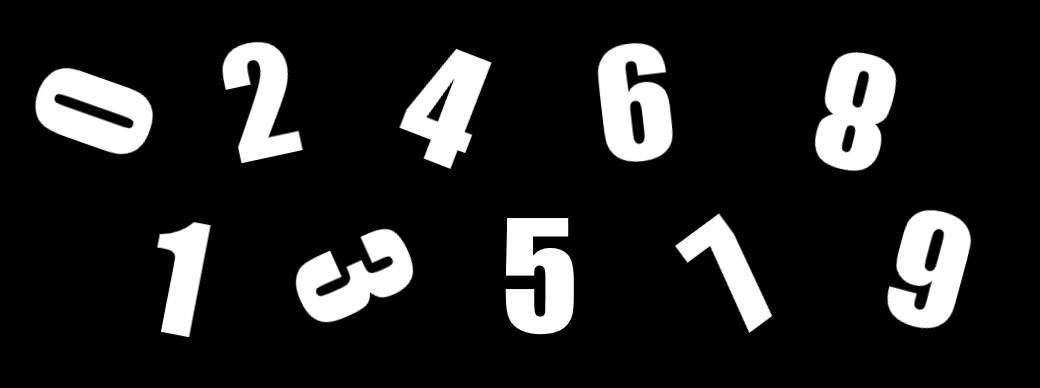# NumbersA simple program that allows a computer or 2 opponents to compete in the "Numbers" game.

### The numbers game is defined as follows

• Each player has two numbers. Both numbers start with a value of 1.
• Goal is to reach a set number (usually 8) by manipulating your number with an opponent's number via addition, subtraction, multiplication, or division
• Each player takes turns modifying their numbers until both player's numbers are the goal number
• A player cannot perform calculations only between their own numbers
• A player cannot modify an opponent's number directly
• Numbers are between 0-9
• Negative numbers are not allowed
• Fractions are not allowed
• In the event that a player performs a calculation that exceeds 9, the rightmost digit is preserved (i.e. 3*6 = 18, player number is 8)

### Example game:

• (pl1: 1|1, pl2: 1|1) Player 1 adds opponent's left number to their left number
• (pl1: 2|1, pl2: 1|1) Player 2 adds opponent's left number to their left number
• (pl1: 2|1, pl2: 3|1) Player 1 multiplies opponent's left number with their right number
• (pl1: 2|3, pl2: 3|1) Player 2 multiples opponent's right number with their left number
• (pl1: 2|1, pl2: 9|1) Player 1 subtracts opponent's right from their right number
• (pl1: 2|8, pl2: 9|1) Player 2 subtracts opponent's right number from their left number
• (pl1: 2|8, pl2: 1|1) Player 1 adds opponent's right number to their left number
• (pl1: 3|8, pl2: 1|1) Player 2 multiplies opponent's right number with their left number
• (pl1: 3|8, pl2: 8|1) Player 1 adds opponent's right number to their left number
• (pl1: 4|8, pl2: 8|1) Player 2 multiplies opponent's right number with their right number
• (pl1: 4|8, pl2: 8|8) Player 2 wins